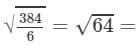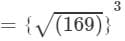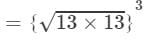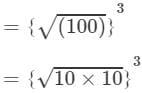# RD Sharma Solutions for Class 8 Math Chapter 4 - Cubes and Cube Roots (Part-2) Notes | Study RD Sharma Solutions for Class 8 Mathematics - Class 8

## Class 8: RD Sharma Solutions for Class 8 Math Chapter 4 - Cubes and Cube Roots (Part-2) Notes | Study RD Sharma Solutions for Class 8 Mathematics - Class 8

The document RD Sharma Solutions for Class 8 Math Chapter 4 - Cubes and Cube Roots (Part-2) Notes | Study RD Sharma Solutions for Class 8 Mathematics - Class 8 is a part of the Class 8 Course RD Sharma Solutions for Class 8 Mathematics.
All you need of Class 8 at this link: Class 8

#### Answer 16: Surface area of a cube is given by:SA=6s2SA=6s2, where s = Side of the cubeFurther, volume of a cube is given by:V=s3V=s3, where s = Side of the cubeIt is given that the surface area of the cube is 384 m2. Therefore, we have:6s2=384⇒s=8 m6s2=384⇒s=3846=64=8 m

Now, volume is given by:
V=s3=83V= 8×8×8 =512 m3V=s3=83⇒V= 8×8×8 =512 m3
Thus, the required volume is 512 m3.

#### ={(169)1/2}3={13}3=13×13×13=2197

(ii) To evaluate the value of the given expression, we can proceed as follows:

{(62+82)1/2}3={(36+64)1/2}3={(100)1/2}3={10}3=10×10×10=1000

#### Answer 19: (i) We have to find the cube of 35 using column method. We have: a=3 and b=5a=3 and b=5

 Column Ia3 Column II3×a2×b3×a2×b Column III3×a×b23×a×b2 Column IVb3 33=27 3×a2×b=3×32×5=135 3×a×b2=3×3×52=225 53=125 +15 +23 + 12 125 42 158 237 42 8 7 5

Thus, cube of 35 is 42875.
(ii) We have to find the cube of 56 using column method. We have: a=5 and b=6a=5 and b=6

 Column Ia3 Column II3×a2×b3×a2×b Column III3×a×b2 Column IVb3 53=125 3×a2×b=3×52×6=450 3×a×b2=3×5×62=540 63=216 +50 +56 + 21 216 175 506 561 175 6 1 6

Thus, cube of 56 is 175616.
(iii) We have to find the cube of 72 using column method. We have: a=7 and b=2a=7 and b=2

 Column Ia3 Column II3×a2×b Column III3×a×b23×a×b2 Column IVb3 73=343 3×a2×b=3×72×2=294 3×a×b2=3×7×22=84 23=8 +30 +8 +0 8 373 302 84 373 2 4 8

Thus, cube of 72 is 373248.